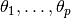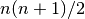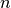# Dependent¶

Dependent is an abstract type designed to store values and attributes of model nodes, including parametersto be simulated via MCMC, functions of the parameters, and likelihood specifications on observed data. It extends the base Variate type with method functions defined for the fields summarized below. Like the type it extends, values are stored in a value field and can be used with method functions that accept Variate type objects.

Since parameter values in the Dependent structure are stored as a scalar or array, objects of this type can be created for model parameters of corresponding dimensions, with the choice between the two being user and application-specific. At one end of the spectrum, a model might be formulated in terms of parameters that are all scalars, with a separate instances of Dependent for each one. At the other end, a formulation might be made in terms of a single parameter array, with one corresponding instance of Dependent. Whether to formulate parameters as scalars or arrays will depend on the application at hand. Array formulations should be considered for parameters and data that have multivariate distributions, or are to be used as such in numeric operations and functions. In other cases, scalar parametrizations may be preferable. Situations in which parameter arrays are often used include the specification of regression coefficients and random effects.

## Declaration¶

abstract Dependent{T} <: Variate{T}

## Fields¶

• value::T : a scalar or array of VariateType values that represent samples from a target distribution.
• symbol::Symbol : an identifying symbol for the node.
• nlink::Integer : number of elements returned by the link method defined on the type. Generally, this will be the number of unique elements in the node. In most cases, nlink will be equal to length(value). However, for some structures, like stochastic covariance matrices, nlink may be smaller.
• monitor::Vector{Int} : indices identifying elements of the value field to include in monitored MCMC sampler output.
• eval::Function : a function for updating the state of the node.
• sources::Vector{Symbol} : symbols of other nodes upon whom the values of this one depends.
• targets::Vector{Symbol} : symbols of Dependent nodes that depend on this one. Elements of targets are topologically sorted so that a given node in the vector is conditionally independent of subsequent nodes, given the previous ones.

## Methods¶

Apply a node-specific inverse-link transformation. In this method, the link transformation is defined to be the identity function. This method may be redefined for subtypes of Dependent to implement different link transformations.

Arguments

• d : a node on which a link() transformation method is defined.
• x : an object to which to apply the inverse-link transformation.
• transform : whether to transform x or assume an identity link.

Value

Returns the inverse-link-transformed version of x.

Apply a node-specific link transformation. In this method, the link transformation is defined to be the identity function. This method function may be redefined for subtypes of Dependent to implement different link transformations.

Arguments

• d : a node on which a link() transformation method is defined.
• x : an object to which to apply the link transformation.
• transform : whether to transform x or assume an identity link.

Value

Returns the link-transformed version of x.
logpdf(d::Dependent, transform::Bool=false)

Evaluate the log-density function for a node. In this method, no density function is assumed for the node, and a constant value of 0 is returned. This method function may be redefined for subtypes of Dependent that have distributional specifications.

Arguments

• d : a node containing values at which to compute the log-density.
• transform : whether to evaluate the log-density on the link-transformed scale.

Value

The resulting numeric value of the log-density.
setmonitor!(d::Dependent, monitor::Bool)
setmonitor!(d::Dependent, monitor::Vector{Int})

Specify node elements to be included in monitored MCMC sampler output.

Arguments

• d : a node whose elements contain sampled MCMC values.
• monitor : a boolean indicating whether all elements are monitored, or a vector of element-wise indices of elements to monitor.

Value

Returns d with its monitor field updated to reflect the specified monitoring.
show(d::Dependent)

Write a text representation of nodal values and attributes to the current output stream.

showall(d::Dependent)

Write a verbose text representation of nodal values and attributes to the current output stream.

# Logical¶

Type Logical inherits the fields and method functions from the Dependent type, and adds the constructors and methods listed below. It is designed for nodes that are deterministic functions of model parameters and data. Stored in the field eval is an anonymous function defined as

function(model::Mamba.Model)


where model contains all model nodes. The function can contain any valid julia expression or code block written in terms of other nodes and data structures. It should return values with which to update the node in the same type as the value field of the node.

## Declaration¶

type Logical{T} <: Dependent{T}

## Fields¶

• value::T : a scalar or array of VariateType values that represent samples from a target distribution.
• symbol::Symbol : an identifying symbol for the node.
• nlink::Integer : number of elements returned by the link method defined on the type.
• monitor::Vector{Int} : indices identifying elements of the value field to include in monitored MCMC sampler output.
• eval::Function : a function for updating values stored in value.
• sources::Vector{Symbol} : symbols of other nodes upon whom the values of this one depends.
• targets::Vector{Symbol} : symbols of Dependent nodes that depend on this one. Elements of targets are topologically sorted so that a given node in the vector is conditionally independent of subsequent nodes, given the previous ones.

## Constructors¶

Logical(expr::Expr, monitor::Union(Bool, Vector{Int})=true)
Logical(d::Integer, expr::Expr, monitor::Union(Bool, Vector{Int})=true)

Construct a Logical object that defines a logical model node.

Arguments

• d : number of dimensions for array nodes.
• expr : a quoted expression or code-block defining the body of the function stored in the eval field.
• monitor : a boolean indicating whether all elements are monitored, or a vector of element-wise indices of elements to monitor.

Value

Returns a Logical{Array{VariateType,d}} if the dimension argument d is specified, and a Logical{VariateType} if not.

Example

See the Model Specification section of the tutorial.

## Methods¶

setinits!(l::Logical, m::Model, ::Any=nothing)

Set initial values for a logical node.

Arguments

• l : a logical node to which to assign initial values.
• m : a model that contains the node.

Value

Returns the result of a call to update!(l, m).
update!(l::Logical, m::Model)

Update the values of a logical node according to its relationship with others in a model.

Arguments

• l : a logical node to update.
• m : a model that contains the node.

Value

Returns the node with its values updated.

# Stochastic¶

Type Stochastic inherits the fields and method functions from the Dependent type, and adds the additional ones listed below. It is designed for model parameters or data that have distributional or likelihood specifications, respectively. Its stochastic relationship to other nodes and data structures is represented by the Distributions structure stored in field distr. Stored in the field eval is an anonymous function defined as

function(model::Mamba.Model)


where model contains all model nodes. The function can contain any valid julia expression or code-block. It should return a single Distributions object for all node elements or a structure of the same type as the node with element-specific Distributions objects.

## Declaration¶

type Stochastic{T} <: Dependent{T}

## Fields¶

• value::T : a scalar or array of VariateType values that represent samples from a target distribution.
• symbol::Symbol : an identifying symbol for the node.
• nlink::Integer : number of elements returned by the link method defined on the type.
• monitor::Vector{Int} : indices identifying elements of the value field to include in monitored MCMC sampler output.
• eval::Function : a function for updating the distr field for the node.
• sources::Vector{Symbol} : symbols of other nodes upon whom the distributional specification for this one depends.
• targets::Vector{Symbol} : symbols of Dependent nodes that depend on this one. Elements of targets are topologically sorted so that a given node in the vector is conditionally independent of subsequent nodes, given the previous ones.
• distr::DistributionStruct : the distributional specification for the node.

## Aliases¶

typealias DistributionStruct Union(Distribution, Array{Distribution})


## Constructors¶

Stochastic(expr::Expr, monitor::Union(Bool, Vector{Int})=true)
Stochastic(d::Integer, expr::Expr, monitor::Union(Bool, Vector{Int})=true)

Construct a Stochastic object that defines a stochastic model node.

Arguments

• d : number of dimensions for array nodes.
• expr : a quoted expression or code-block defining the body of the function stored in the eval field.
• monitor : a boolean indicating whether all elements are monitored, or a vector of element-wise indices of elements to monitor.

Value

Returns a Stochastic{Array{VariateType,d}} if the dimension argument d is specified, and a Stochastic{VariateType} if not.

Example

See the Model Specification section of the tutorial.

## Methods¶

insupport(s::Stochastic)

Check whether stochastic node values are within the support of its distribution.

Arguments

• s : a stochastic node on which to perform the check.

Value

Returns true if all values are within the support, and false otherwise.
invlink(s::Stochastic, x, transform::Bool=true)

Apply an inverse-link transformation to map transformed values back to the original distributional scale of a stochastic node.

Arguments

• s : a stochastic node on which a link() transformation method is defined.
• x : an object to which to apply the inverse-link transformation.
• transform : whether to transform x or assume an identity link.

Value

Returns the inverse-link-transformed version of x.
link(s::Stochastic, x, transform::Bool=true)

Apply a link transformation to map values in a constrained distributional support to an unconstrained space. Supports for continuous, univariate distributions and positive-definite matrix distributions (Wishart or inverse-Wishart) are transformed as follows:

• Lower and upper bounded: scaled and shifted to the unit interval and logit-transformed.
• Lower bounded: shifted to zero and log-transformed.
• Upper bounded: scaled by -1, shifted to zero, and log-transformed.
• Positive-definite matrix: compute the (upper-triangular) Cholesky decomposition, and return its log-transformed diagonal elements prepended to the remaining upper-triangular part as a vector of length, whereis the matrix dimension.

Arguments

• s : a stochastic node on which a link() transformation method is defined.
• x : an object to which to apply the link transformation.
• transform : whether to transform x or assume an identity link.

Value

Returns the link-transformed version of x.
logpdf(s::MCMStochastic, transform::Bool=false)

Evaluate the log-density function for a stochastic node.

Arguments

• s : a stochastic node containing values at which to compute the log-density.
• transform : whether to evaluate the log-density on the link-transformed scale.

Value

The resulting numeric value of the log-density.
setinits!(s::Stochastic, m::Model, x=nothing)

Set initial values for a stochastic node.

Arguments

• s : a stochastic node to which to assign initial values.
• m : a model that contains the node.
• x : values to assign to the node.

Value

Returns the node with its assigned initial values.
update!(s::Stochastic, m::Model)

Update the values of a stochastic node according to its relationship with others in a model.

Arguments

• s : a stochastic node to update.
• m : a model that contains the node.

Value

Returns the node with its values updated.